I’ll admit that I’m terrible with regular expressions (REGEX). That’s why I asked Don Wise to write a series of guest blog posts on the basics of regex and how to use them. Fortunately, due to my work on the Tableau Community Forums and watching people like Don, I’ve been able to pick up a few of the basics over time and I’ve created a small library of some of the more useful regex techniques. So, in this blog, I want to share five of the most handy Tableau regex techniques I’ve encountered.

Note: I won’t be going into detail on how each of these works because, as I’ve noted, I’m terrible with Regex. But hopefully, like me, you’ll find opportunities to plug-and-play these in your own work.

Formatting numbers in a specific way can be quite a struggle, particularly when you have a mix of different formats. For example, let’s say your data set looks something like this: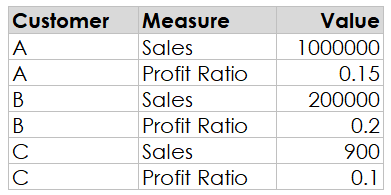We’d ideally want to display the profit ratios as percentages and the sales amounts as currency, but this can be a bit tricky since both values are in a single column.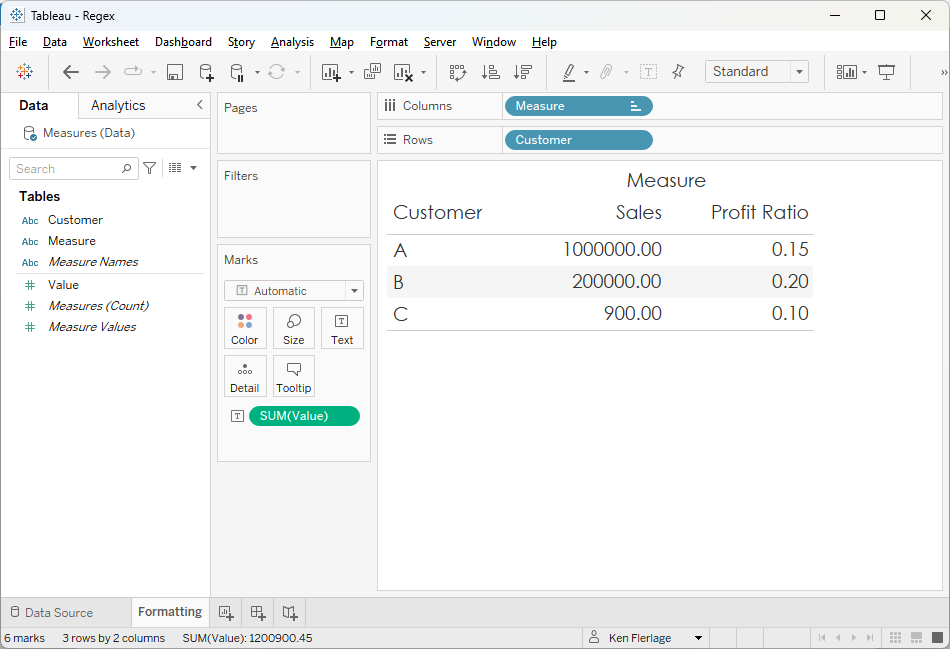My go-to solution for this problem is to create separate measures then format them individually as detailed by Kevin’s blog, Dynamically Control Formatting Using Multiple Calculations. But there are some situations where this method doesn’t work, and you’re forced to format the values as strings. Formatting a number as a percentage is pretty easy…

Value Formatted

// Format profit ratio as % and sales as currency.

IF MAX([Measure])="Profit Ratio" THEN

STR(SUM([Value])*100) + "%"

END

But, when formatting large numbers, I like to add thousands separators. That’s not so easy with basic formulas. Fortunately, there’s a regex for that!!

Value Formatted

// Format profit ratio as % and sales as currency.

IF MAX([Measure])="Profit Ratio" THEN

STR(SUM([Value])*100) + "%"

ELSE

"\$" + REGEXP_REPLACE(STR(SUM([Value])), "\d{1,3}(?=(\d{3})+(?!\d))", "\$0,")

END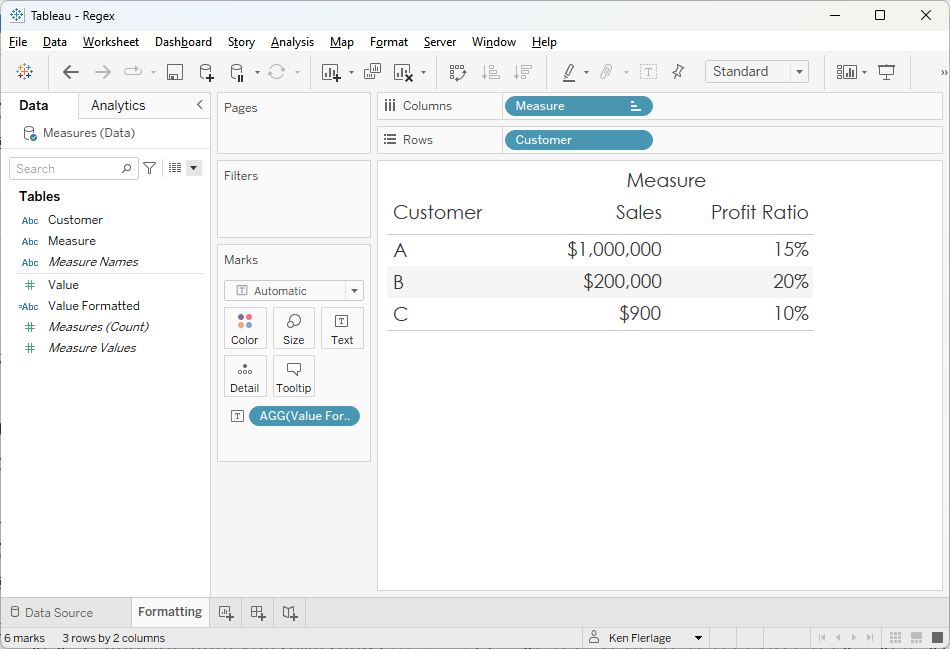2) Extract Value from JSON

In recent years, JSON has become a ubiquitous data format. There are many database platforms, such as MongoDB, which are completely based on the format. And most other database platforms now have the ability to handle JSON data. The result of this is that we often see JSON data getting mixed in with simple tabular data structures. Imagine you have the following data where Name, Age, and DOB are in a single field of JSON data.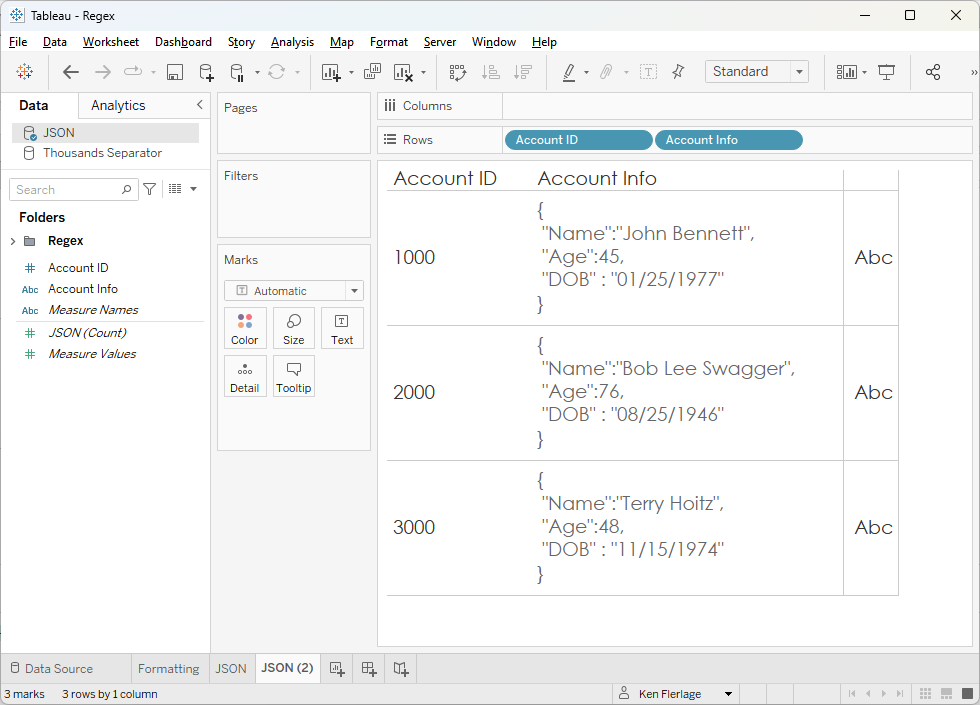Bonus points for anyone who can tell me what these three accounts have in common!! 😉

We could extract each of these fields using a combination of string parsing functions like MID, FIND, etc. but regex provides a much simpler technique. We can use a word capture, simply passing in the JSON key. For example, to get the Name, we’d use:

Name

// Extract name from the JSON field

REGEXP_EXTRACT([Account Info], '"Name":"((\\"|[^"])*)"')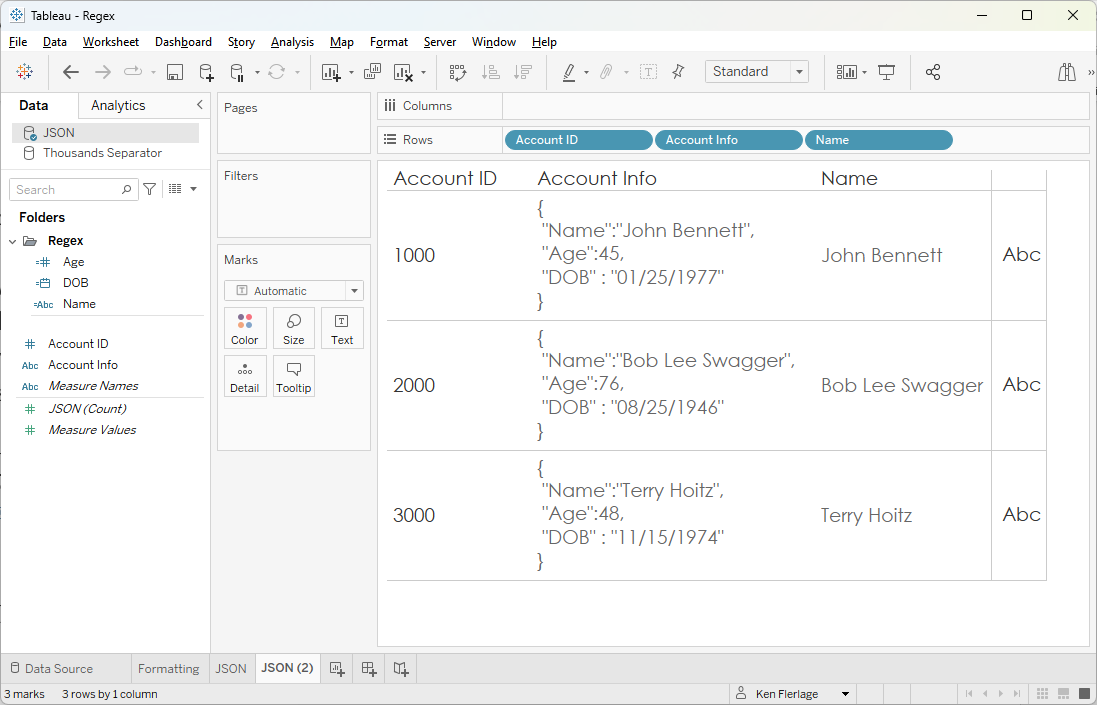So, to get Age, we could just use the following, correct?

Age

// Extract age from the JSON field

REGEXP_EXTRACT([Account Info], '"Age":"((\\"|[^"])*)"')

Unfortunately, no. The age in the JSON data is a number so it’s not quoted like the strings. So, we’d need to remove the double quotes that appear after the colon and before the final single quote.

Age

// Extract age from the JSON field

REGEXP_EXTRACT([Account Info], '"Age":((\\"|[^"])*)')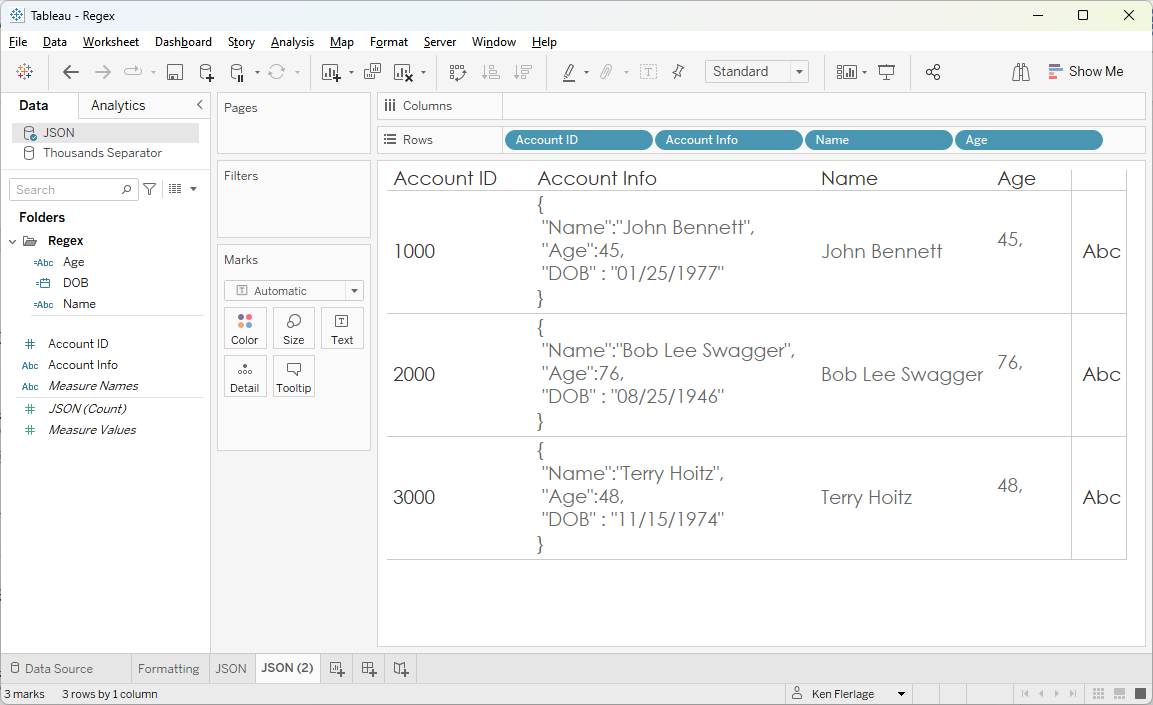But this has a couple of problems. First, it grabs the comma after the age, which we don’t want. Second, we want this to be a number, not a string. To address the comma, we can simply add that before the final single quote. And, to make it a number, we can wrap the entire statement with INT.

Age

// Extract age from the JSON field

INT(REGEXP_EXTRACT([Account Info], '"Age":((\\"|[^"])*),'))

Okay, so DOB should be easy, right? It has double quotes like Name, so we should be able to do the following:

DOB

// Extract DOB from the JSON field

REGEXP_EXTRACT([Account Info], '"DOB":"((\\"|[^"])*)"')

Nope! Notice that DOB has a space before and after the colon, so we’ll need to add those spaces to our pattern. Additionally, since this is a date, we’ll want to convert it to a date.

DOB

// Extract DOB from the JSON field

DATE(REGEXP_EXTRACT([Account Info], '"DOB" : "((\\"|[^"])*)"'))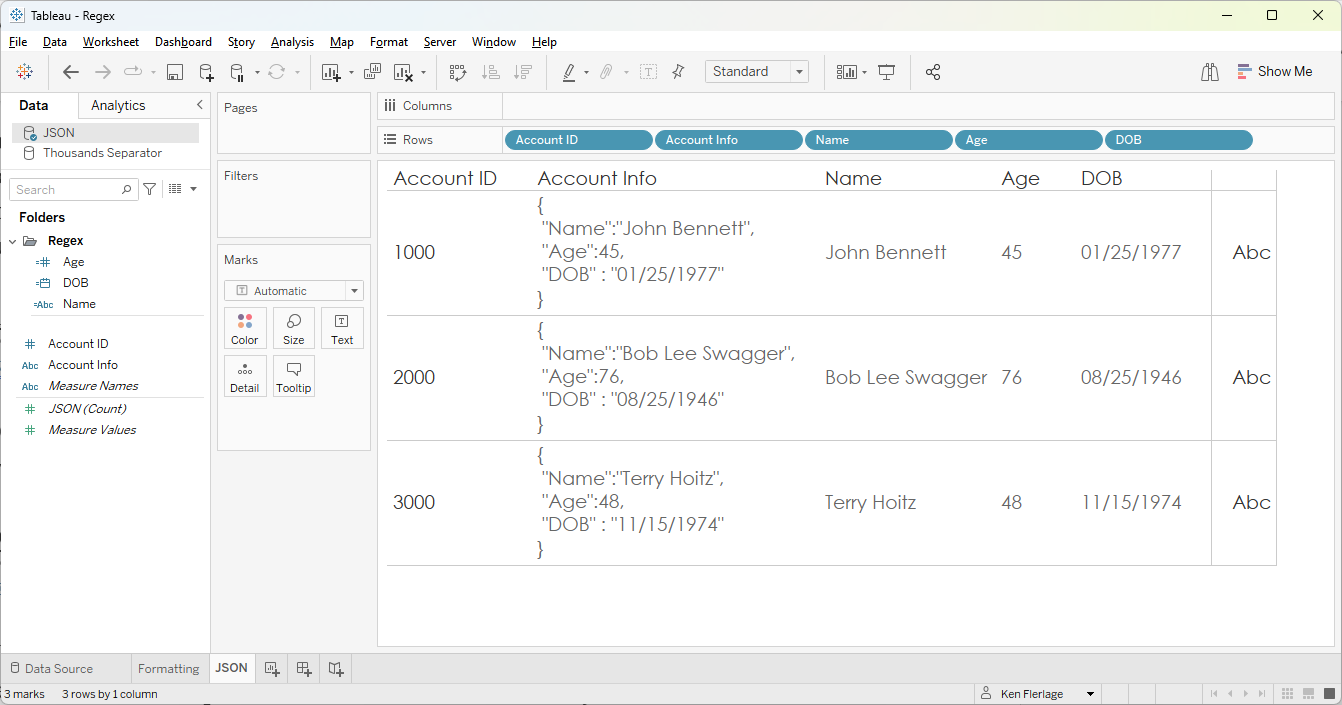Note: There are a bunch of different ways to do this using regex and I’ll admit that I’m not sure if this is the best. But it works! Big thanks to Dovi Lilling from whom I learned this technique on the following forums post: Parse Fields Containing JSON Data.

3) Remove Special Characters

Sometimes, for whatever reason “invisible” characters will find their way into our data. And when it does, it can wreak havoc. Take the following data set, for example.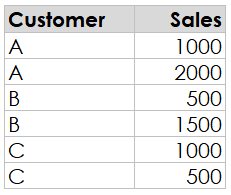If we create a bar chart in Tableau, we should see a total of 3000 for A, 2000 for B, and 1500 for C, correct? But, instead, we see this: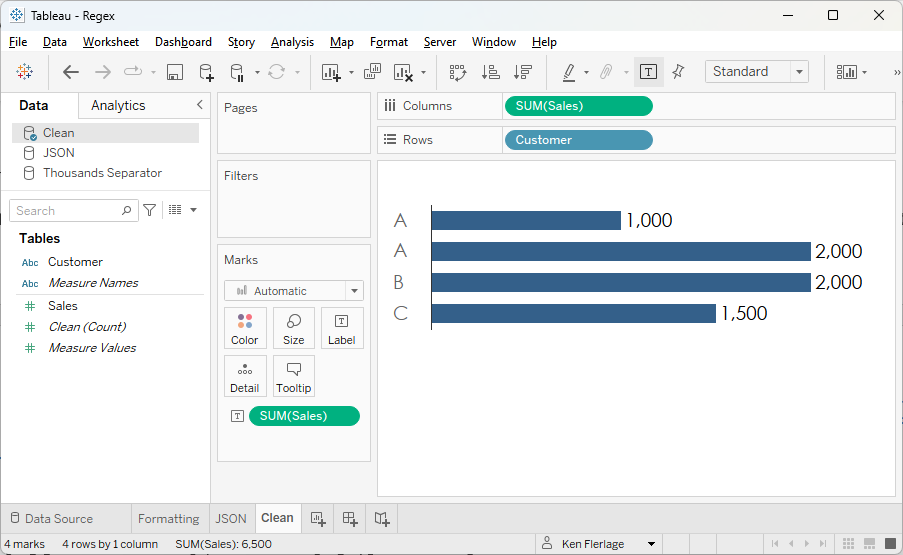What on earth is happening here? It looks like Tableau has aggregated B and C, but not A. When you see this, many times the cause is an “invisible” character—one that is there but doesn’t actually show on screen. To test this theory, we can create a calculated field to get the length of the string.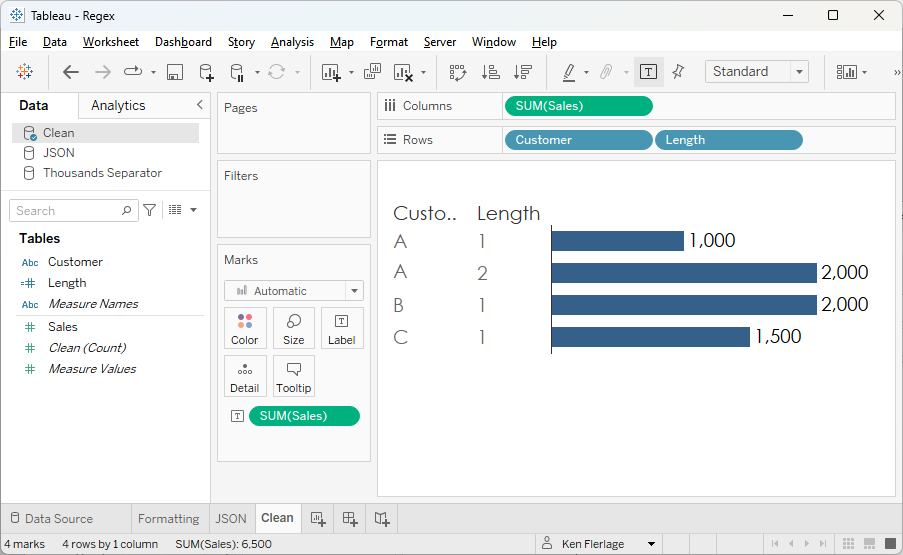We can see that the second “A” has a length of 2, confirming our suspicion. In some cases, this may be a valid value, but in many cases, it is not and needs to be cleaned up. You should always get the issue corrected in the source system or you’ll inevitably have to deal with it again in the future. But we can use regex as a temporary workaround.

Customer Clean

// Remove any non-alphanumeric characters from customer.

REGEXP_REPLACE([Customer], "[^a-zA-Z0-9]", "")

This will remove any character that is not either an upper or lower case letter or 0 through 9. If you want to keep some special characters such as spaces or periods, you can simply add those to the end of the pattern:

Customer Clean

// Remove any non-alphanumeric characters from customer.

REGEXP_REPLACE([Customer], "[^a-zA-Z0-9 .]", "")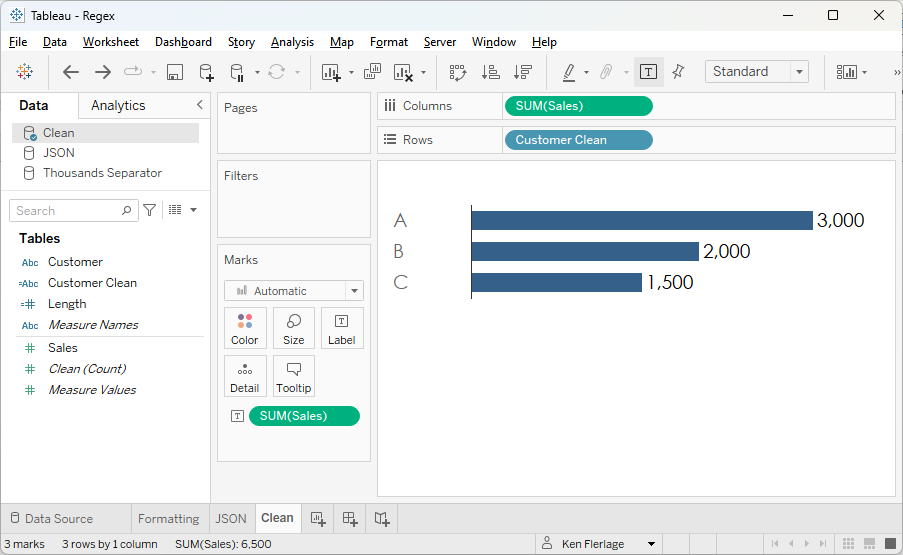Just be careful here—you don’t want to inadvertently remove something you need.

4) Find a String Between Two Other Strings

Unfortunately for us, data isn’t always as clean as we like. Sometimes important information is locked up in some sort of free-text field. But, if there is some structure to that text, we might be able to extract that information. Take the following example.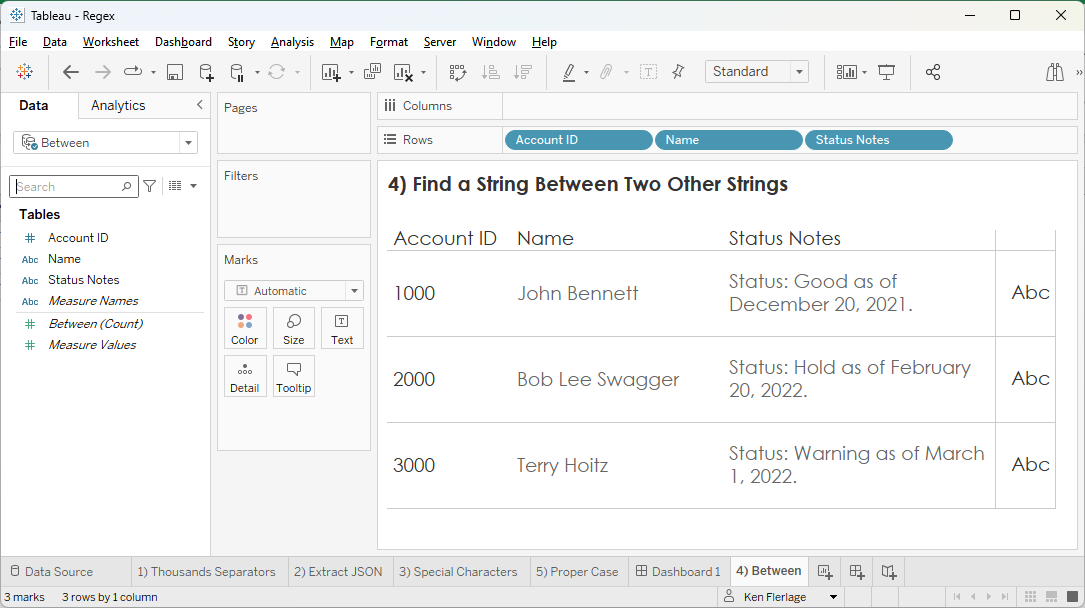It would be great to be able to extract the status (Good, Hold, Warning) from the Status Notes field. In the example above, the status is always preceded by the text, “Status: “ and followed by the text, “ as of”. If we are sure that the notes always follow this same basic structure, then we can use regex to extract just that status value.

Status

// Extract the status from Status Notes.

TRIM(REGEXP_EXTRACT([Status Notes], "((?<=Status:).*?(?=as of))"))

Just replace “Status” and “as of” with your preceding and following text.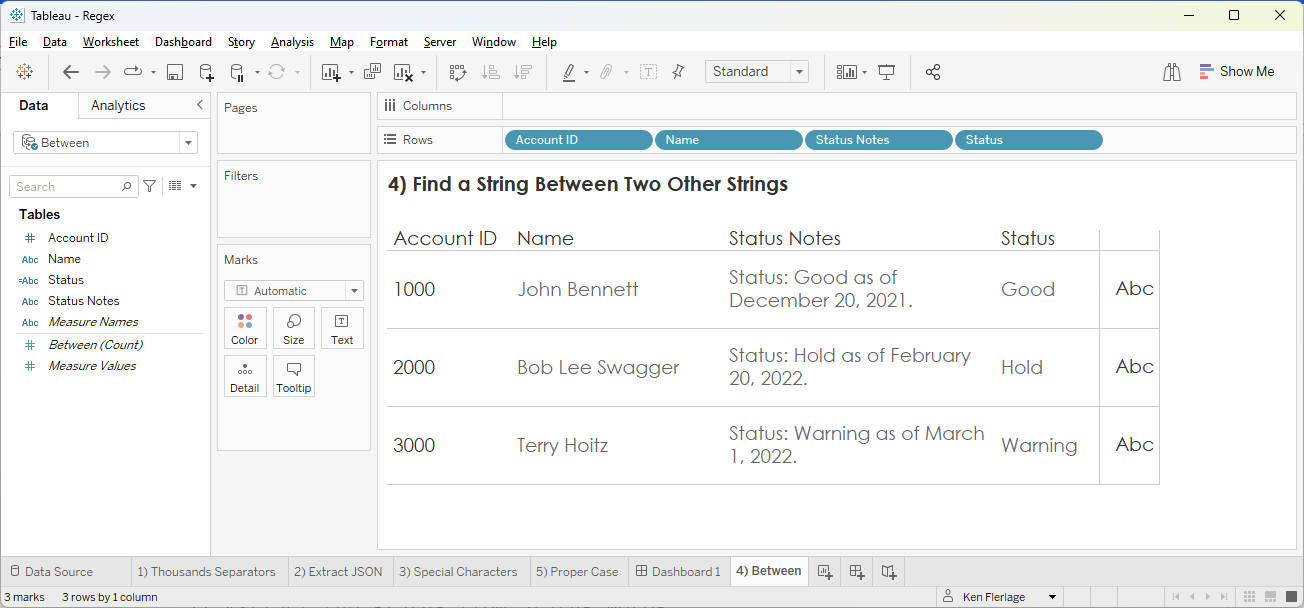5) Proper Case

Sometimes those data entry people are lazy and enter data in all upper case, all lower case, or worst of all, mixed cases, like this: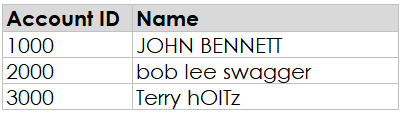This always looks sloppy to me. Using consistent casing such as all upper or all lower case is an improvement, but I still think it’s best to use Proper casing—the first letter of each word is capitalized.

If you’re lucky enough to be on version 2022.4 of Tableau (still very new as of the writing of this blog), then you may know that they’ve introduced a new PROPER function to take care of this for you. But many of you may not be on 2022.4 yet. Luckily, there’s a regex for that. The credit for this one goes to Naomi Estrin for her answer to the following forums question: Proper Case

Name Proper

// Proper case the name.

REGEXP_REPLACE(REGEXP_REPLACE(REGEXP_REPLACE(REGEXP_REPLACE(REGEXP_REPLACE(REGEXP_REPLACE(REGEXP_REPLACE(REGEXP_REPLACE(REGEXP_REPLACE(REGEXP_REPLACE(REGEXP_REPLACE(REGEXP_REPLACE(REGEXP_REPLACE(REGEXP_REPLACE(REGEXP_REPLACE(REGEXP_REPLACE(REGEXP_REPLACE(REGEXP_REPLACE(REGEXP_REPLACE(REGEXP_REPLACE(REGEXP_REPLACE(REGEXP_REPLACE(REGEXP_REPLACE(REGEXP_REPLACE(REGEXP_REPLACE(REGEXP_REPLACE(REGEXP_REPLACE(REGEXP_REPLACE(REGEXP_REPLACE(REGEXP_REPLACE(REGEXP_REPLACE(REGEXP_REPLACE(REGEXP_REPLACE(REGEXP_REPLACE(REGEXP_REPLACE(REGEXP_REPLACE(REGEXP_REPLACE(REGEXP_REPLACE(REGEXP_REPLACE(REGEXP_REPLACE(REGEXP_REPLACE(REGEXP_REPLACE(REGEXP_REPLACE(REGEXP_REPLACE(REGEXP_REPLACE(REGEXP_REPLACE(REGEXP_REPLACE(REGEXP_REPLACE(REGEXP_REPLACE(REGEXP_REPLACE(REGEXP_REPLACE(REGEXP_REPLACE(LOWER([Name]), "^a", "A"), "^b", "B"), "^c", "C"), "^d", "D"), "^e", "E"), "^f", "F"), "^g", "G"), "^h", "H"), "^i", "I"), "^j", "J"), "^k", "K"), "^l", "L"), "^m", "M"), "^n", "N"), "^o", "O"), "^p", "P"), "^q", "Q"), "^r", "R"), "^s", "S"), "^t", "T"), "^u", "U"), "^v", "V"), "^w", "W"), "^x", "X"), "^y", "Y"), "^z", "Z"), " a", " A"), " b", " B"), " c", " C"), " d", " D"), " e", " E"), " f", " F"), " g", " G"), " h", " H"), " i", " I"), " j", " J"), " k", " K"), " l", " L"), " m", " M"), " n", " N"), " o", " O"), " p", " P"), " q", " Q"), " r", " R"), " s", " S"), " t", " T"), " u", " U"), " v", " V"), " w", " W"), " x", " X"), " y", " Y"), " z", " Z")

That’s a long one, but it works beautifully!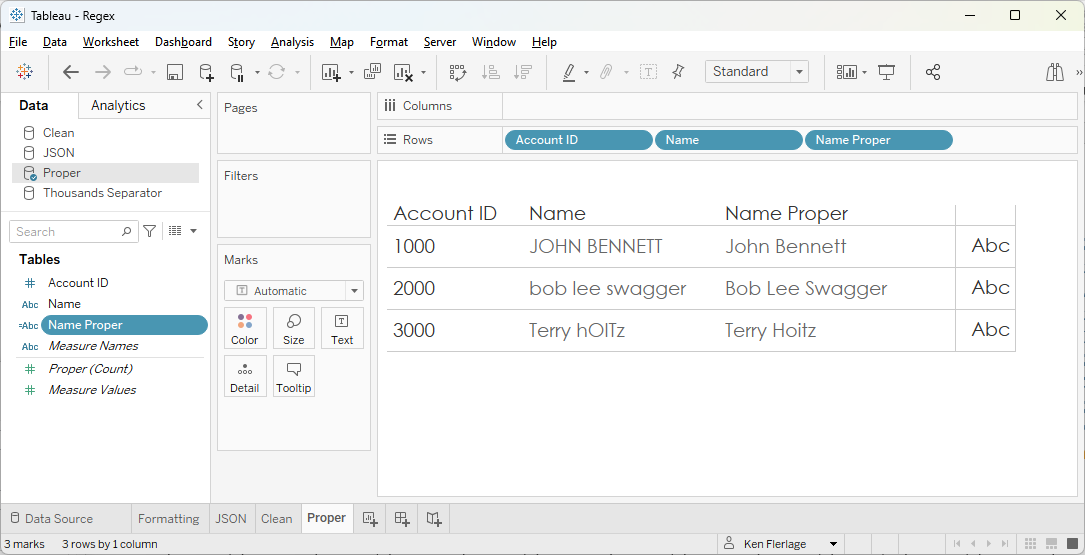Wrap-Up

So there you have it—five incredibly useful Tableau Regex techniques. Thanks for reading. I hope that you’ll be able to apply some of these in your own work. The sample workbook used in this post is available on Tableau Public if you'd like to interact with it: Regex Examples

Ken Flerlage, January 16, 20231.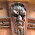I really liked the "Proper" substitution in #5! I found that I could do it in half the number of commands by using "(^| )a", "\$1A") in front of each letter. Not sure if that will speed up any processing, though...

```
REGEXP_REPLACE(REGEXP_REPLACE(REGEXP_REPLACE(REGEXP_REPLACE(
REGEXP_REPLACE(REGEXP_REPLACE(REGEXP_REPLACE(REGEXP_REPLACE(REGEXP_REPLACE(
REGEXP_REPLACE(REGEXP_REPLACE(REGEXP_REPLACE(REGEXP_REPLACE(REGEXP_REPLACE(
REGEXP_REPLACE(REGEXP_REPLACE(REGEXP_REPLACE(REGEXP_REPLACE(REGEXP_REPLACE(
REGEXP_REPLACE(REGEXP_REPLACE(REGEXP_REPLACE(REGEXP_REPLACE(REGEXP_REPLACE(
REGEXP_REPLACE(REGEXP_REPLACE(LOWER([Name]), "(^| )a", "\$1A"), "(^| )b", "\$1B"),
"(^| )c", "\$1C"), "(^| )d", "\$1D"), "(^| )e", "\$1E"), "(^| )f", "\$1F"), "(^| )g", "\$1G"), "(^| )h", "\$1H"), "(^| )i", "\$1I"),
"(^| )j", "\$1J"), "(^| )k", "\$1K"), "(^| )l", "\$1L"), "(^| )m", "\$1M"), "(^| )n", "\$1N"), "(^| )o", "\$1O"), "(^| )p", "\$1P"),
"(^| )q", "\$1Q"), "(^| )r", "\$1R"), "(^| )s", "\$1S"), "(^| )t", "\$1T"), "(^| )u", "\$1U"), "(^| )v", "\$1V"), "(^| )w", "\$1W"),
"(^| )x", "\$1X"), "(^| )y", "\$1Y"), "(^| )z", "\$1Z")
```

1.Nice! Thank you!

2.In the first example, is the field "MEASURE" already a dimension in the data set with a unique row for each customer and measure? Otherwise I do not understand how you are getting multiple measures to show in different columns.

Thank you.

1.Yes. The data looks exactly like the screenshot shared--3 columns and 6 rows.

3.I'm trying to use REGEXP_REPLACE to format different labels (quantity with thousands separator, \$ amount with thousands separator, and %). I've used the syntax for the second "Value Formatted" example, and mine is not turning out correctly.
Below is my syntax:
CASE [Parameters].[Choose Measure Type]
WHEN 1 THEN REGEXP_REPLACE(STR(ROUND([Choose Measure Type], 0)),
"\d{1,3}(?=(\d{3})+(?!\d))", "0,")
WHEN 2 THEN '\$' +
(REGEXP_REPLACE(STR(ROUND([Choose Measure Type], 0)), "\d{1,3}(?=(\d{3})+(?!\d))", '\$0,'))
WHEN 3 THEN STR(ROUND(ROUND([Choose Measure Type], 4)*100, 2)) + '%'
END
When I place this field in the Label Marks Card, my quantity labels are showing as "0,156" when the true value is "56,156". The \$ amount labels are showing as "\$\$0,\$0,642" where the actual value is "\$219,915,642". However, the % rate is displaying perfectly. Please advise!!